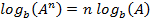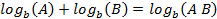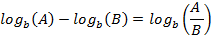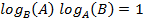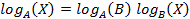Derivative of a Logarithm

DERIVATION

We start with the function for the logarithm and calculate a change in “y” caused by a change in “x” as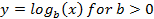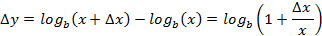where we have defined a new variable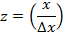The derivative operation for the logarithm function iswhere we have defined the new variable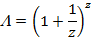Recalling the binominal theorem, we can write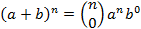+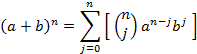where the “binominal coefficients” are the combinatorials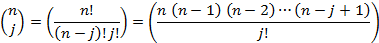noting the last expression above has “j” terms in the numerator.  But in any event using this expression we can writeAnd the limit can be expressed asThe derivative of the logarithm is thus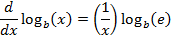or for the special case of the natural logarithm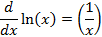REVIEW OF LOGARITHMIC FORMULAE

A logarithm is simply an exponent.   If we begin with the following expression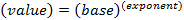then we can define the logarithm as followsIn simple parlance, we say that the logarithm is that exponent which when applied to a base, yields a particular value.

A trivial result from the definition of a logarithm gives us the following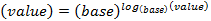or simply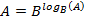The consequences of this simple definition are several, as follows